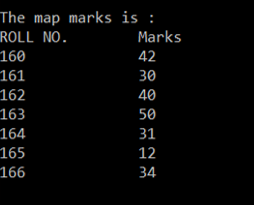AWS Global Infrastructure

### Programming & Frameworks

Topics Covered
• C Programming and Data Structures (41 Blogs)
• Comprehensive Java Course (2 Blogs)
• Java/J2EE and SOA (324 Blogs)
• Spring Framework (8 Blogs)
SEE MORE

# What are Maps in C++ and how to implement it?

Published on Jul 31,2019 2.3K ViewsWhat are Maps in C++ and how to implement it?

Associative containers that store elements in a mapped fashion are called Maps. All the elements in a map are stored in a key-value pair where each key is unique. Sorting is done with the help of keys and the values are associated with each key. Values can be inserted and deleted as and when required.

Let’s get started.

## What are Maps in C++

Maps in C++ are associative containers that store elements formed by a combination of a key value and a mapped value.

Consider the example:

 Keys(Roll NO) Names 16030141001 Akash 16030141002 Yuvraj 16030141003 Ashish 16030141004 Arun

The above example shows a key and value pair. The roll number is the key and each student has a different roll number, hence unique key representing them.

Syntax: map<key_type , value_type> map_name;

This is a basic syntax for creating a map in C++. We have a key value of type key_type and a value associated with the key of the type value_type. When we enter the values, they should be entered in a pair and we cannot enter them one by one.

Moving ahead in C++ Maps, let’s look at the different functions in Maps.

## Functions in Maps

There are many functions associated with maps. They are,

• begin()
• end()
• size()
• max_size()
• empty()
• enter()
• delete()
• insert()
• clear()

Here’s a code for C++ map creation:

```#include <iostream>
#include <iterator>
#include

<map>

using namespace std;

int main()
{
map<int, int> marks;
marks.insert(pair<int, int>(160, 42));
marks.insert(pair<int, int>(161, 30));
marks.insert(pair<int, int>(162, 40));
marks.insert(pair<int, int>(163, 50));
marks.insert(pair<int, int>(164, 31));
marks.insert(pair<int, int>(165, 12));
marks.insert(pair<int, int>(166, 34));

map<int, int>::iterator itr;
cout << "nThe map marks is : n";
cout << "ROLL NO.tMarksn";
for (itr =  marks.begin(); itr !=  marks.end(); ++itr) {
cout  << itr->first
<< "t   t" << itr->second << 'n';
}
cout << endl;
return 0;
}```

## Output:## Explanation:

In the above program, we created a map. We have to include different header files for creating a map. We then create the map container.

`map<int,int> marks;`

Here we create a map named marks, the key and value will be of type int. The container is empty at the start.
We then call the insert function to insert key and value pair. We then create an iterator for the map called iter. We use it inside a for loop till we encounter the last pair in the map. We print keys first and then the value.

## Map Example: How to implement Maps

There are many other functions like the erase function. Here’s the code:

```#include <iostream>
#include <iterator>
#include

<map>
using namespace std;

int main()
{
map<int, int> marks;
marks.insert(pair<int, int>(160, 42));
marks.insert(pair<int, int>(161, 30));
marks.insert(pair<int, int>(162, 40));
marks.insert(pair<int, int>(163, 50));
marks.insert(pair<int, int>(164, 31));
marks.insert(pair<int, int>(165, 12));
marks.insert(pair<int, int>(166, 34));

map<int, int>::iterator itr;
cout << "nThe map marks is : n";
cout << "ROLL NO.tMarksn";
for (itr =  marks.begin(); itr !=  marks.end(); ++itr) {
cout  << itr->first
<< "t   t" << itr->second << 'n';
}
cout << endl;
int num;
num = marks.erase(164);
cout << "nmarks.erase(164) : ";
cout << num << " removed n";
cout << "tROLL NO. tMarksn";
for (itr = marks.begin(); itr != marks.end(); ++itr) {
cout << 't' << itr->first
<< 't' << itr->second << 'n';
}
return 0;
}
```

The only thing we do is call marks.erase(164) to delete the element with 164 as its key or roll number. Remaining part of the code is the same.

Similarly, we can do this with all the other functions provided by the map

Thus we have come to an end of this article on ‘Maps in C++’. If you wish to learn more, check out the Java Training by Edureka, a trusted online learning company. Edureka’s Java J2EE and SOA training and certification course are designed to train you for both core and advanced Java concepts along with various Java frameworks like Hibernate & Spring.

Got a question for us? Please mention it in the comments section of the “Maps in C++”  blog and we will get back to you as soon as possible.REGISTER FOR FREE WEBINARThank you for registering Join Edureka Meetup community for 100+ Free Webinars each month